# Microsoft Excel Computers Week 4 MICROSOFT EXCEL VOCABULARY

• Slides: 16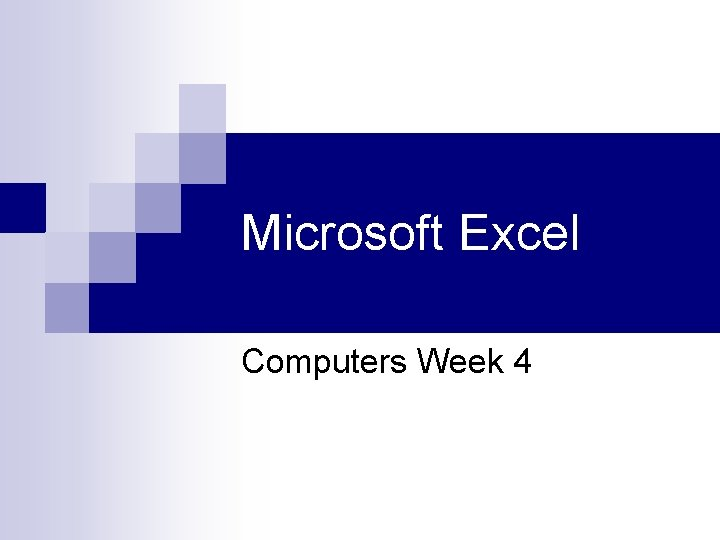Microsoft Excel Computers Week 4Pie Charts10. Finishing In the footer, type Activity 6, press enter and type your name. Don’t forget to print preview to double check that your chart is just the way you want it. Go to File and select Save. Name the file Excel 1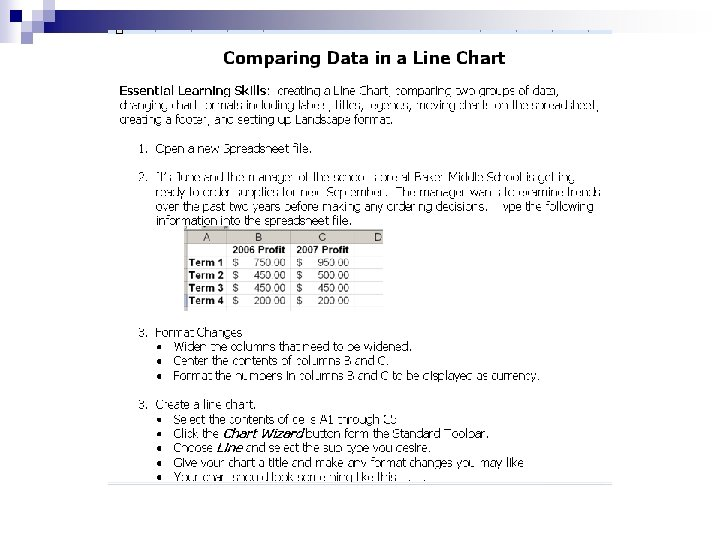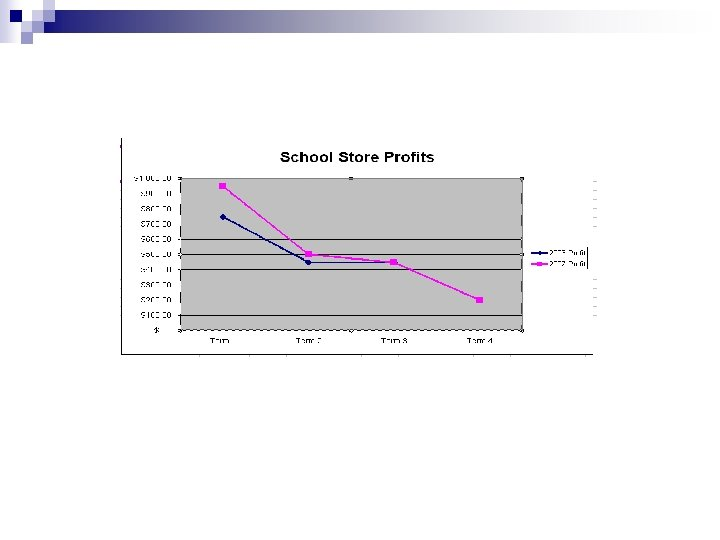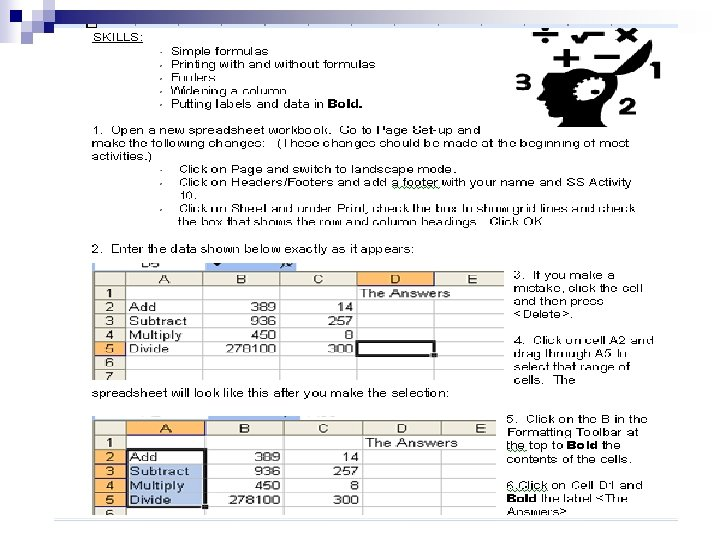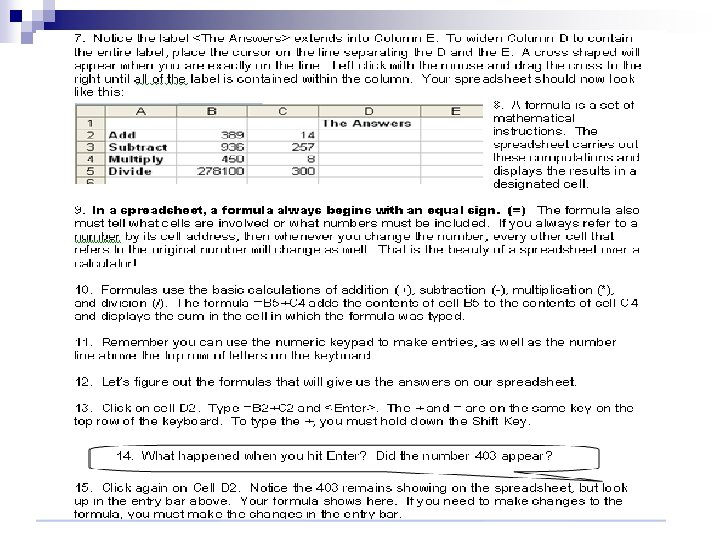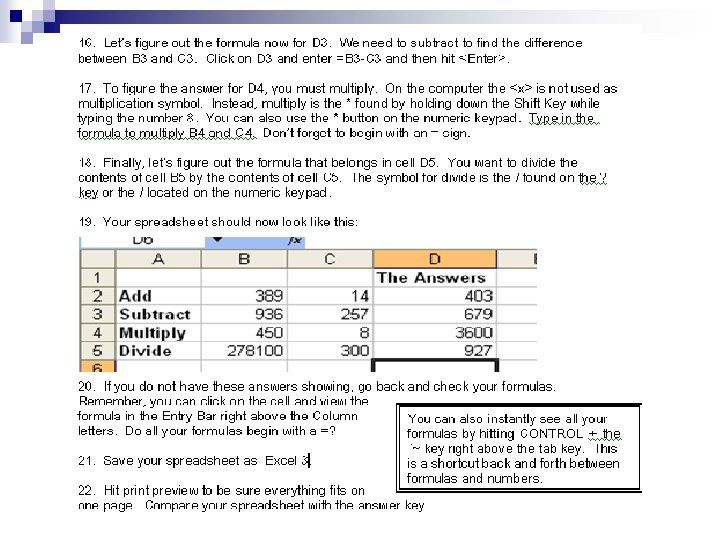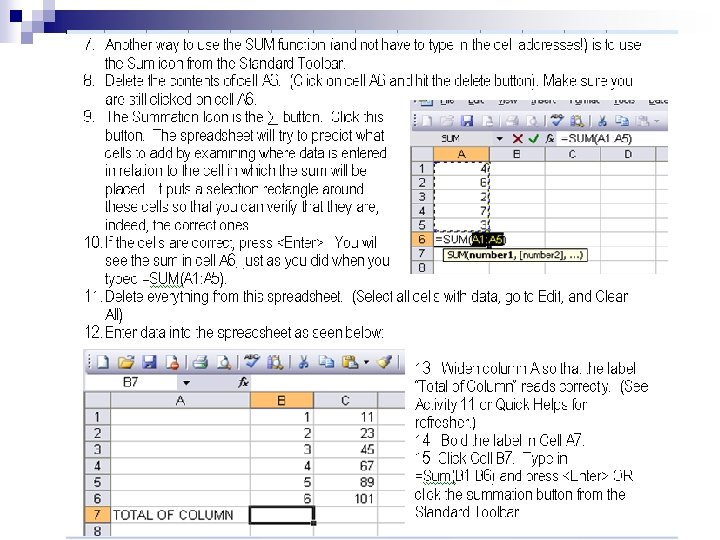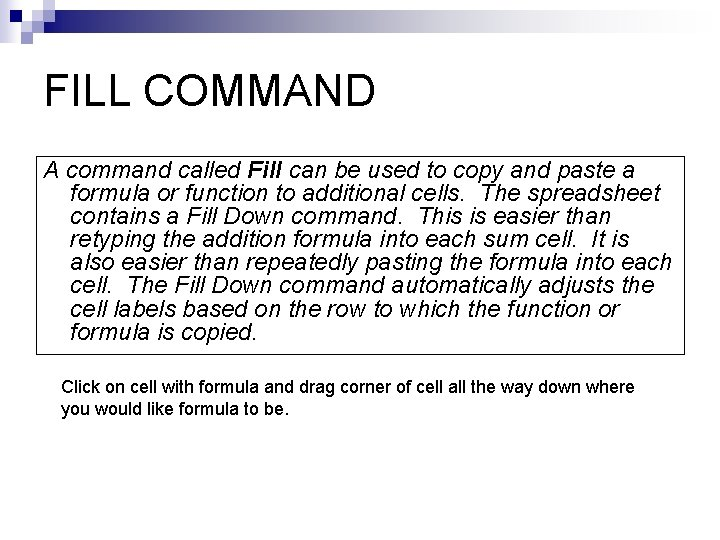FILL COMMAND A command called Fill can be used to copy and paste a formula or function to additional cells. The spreadsheet contains a Fill Down command. This is easier than retyping the addition formula into each sum cell. It is also easier than repeatedly pasting the formula into each cell. The Fill Down command automatically adjusts the cell labels based on the row to which the function or formula is copied. Click on cell with formula and drag corner of cell all the way down where you would like formula to be.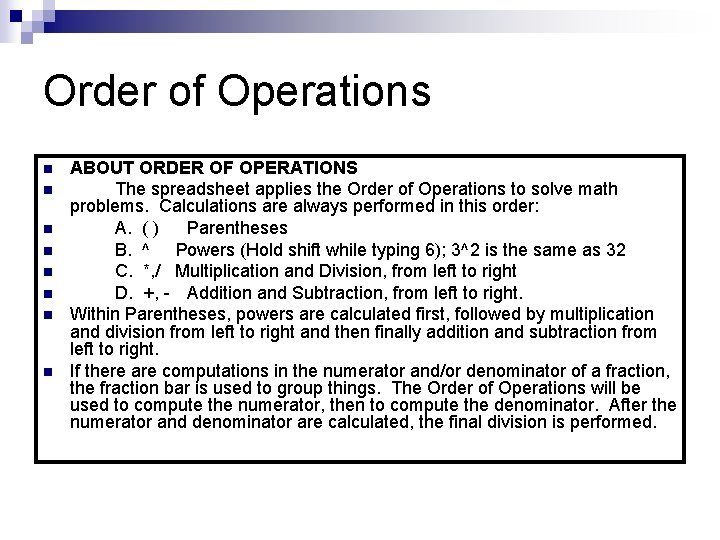Order of Operations n n n n ABOUT ORDER OF OPERATIONS The spreadsheet applies the Order of Operations to solve math problems. Calculations are always performed in this order: A. ( ) Parentheses B. ^ Powers (Hold shift while typing 6); 3^2 is the same as 32 C. *, / Multiplication and Division, from left to right D. +, - Addition and Subtraction, from left to right. Within Parentheses, powers are calculated first, followed by multiplication and division from left to right and then finally addition and subtraction from left to right. If there are computations in the numerator and/or denominator of a fraction, the fraction bar is used to group things. The Order of Operations will be used to compute the numerator, then to compute the denominator. After the numerator and denominator are calculated, the final division is performed.Homework